# Summary Stats Data and Models

ISBN-10 1292022450 ISBN-13 9781292022451
126 Flashcards & Notes
3 Students

# Remember faster, study better. Scientifically proven.## This is the summary of the book "Stats Data and Models". The author(s) of the book is/are Richard D De Veaux. The ISBN of the book is 9781292022451 or 1292022450. This summary is written by students who study efficient with the Study Tool of Study Smart With Chris.

PREMIUM summaries are quality controlled, selected summaries prepared for you to help you achieve your study goals faster!• ## 1 Stats starts here

• What is statistics?

A way of resigning, along with a collection of tools and methods, designed to help us understand the world.

• What are statistics?

particular calculations made from data

• What are data?

Data are values along with their context.

• Where is statistics about?

Statistics is about variation.

• What is one of the interesting chalanges of Statistics?

Here can be more than one right answer.

• ## 2.1 But what are data?

• What do data contain?

Who, what, when, where (and if possible) Why. Often we add How to the list as well.

• What do the 'data questions' tell us?

What the context for the data values is.

• What items do you need at least to have data?

Who and what

• ## 2.2 Data Tables

• Why do we make data tables?

To organize the values and make the context of the data clear.

• ## 2.3 who

• What do rows of a data table correspond with?

Individual cases about Whom (or which- if they're not people) we record some characteristics.

• What are respondents?

Individuals who answer a survey.

• What are subjects?

People on whom we experiment.

• What are participants?

People on whom we experiment.

• What are experimental units?

Animals,  websites or other inanimate subjects on which we experiment.

• What are records?

Rows in a database

• What is another word for record?

Cases

• What is a sample?

Cases selected from some larger population.

• What does 'representative' mean?

The sample of a population is able to generalize  the larger population.

• ## 2.4 What and why

• What are variables?

Characteristics recorded about each individual.

• How do you really understand your variables?

Think about what you want to know.

• What do units tell you?

How each value has been measured.

• What is a categorial variable?

A variable that names categories and answers questions about how cases fall into those categories.

• What is a quantitative variable?

A measured variable with units answers questions about the quantity of what is measured.

• What should you do when it isn't clear if a variable is categorial or quantitive?

Think about Why you are looking at it and what you want it to tell you.

Read the full summary
This summary. +380.000 other summaries. A unique study tool. A rehearsal system for this summary. Studycoaching with videos.

### Latest added flashcards

Why are we speaking of a standard error in CLT?
Because we estimate the standard deviation of the sampling distribution model from the data.
What do we need to model the sampling distribution of ?
A random sample of quantitative data.
A true population standard deviation,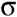.
When is the CLT close tho the normal?
The larger the sample used, the more closely the Normal approximates the sampling distribution for the mean.
What is the shape from a CLT looks like?
The shape of the sampling distribution is approximately Normal as long as the sample size is large enough.
How do we supposed to know ?
We estimate the population parameterwith s, the sample standard deviation based on the data. The resulting standard error is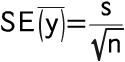What is meant by CLT?
Central limit theorem.
What are conditions for the central limit theorem?
The shape of the sampling distribution is approximately Normal as long as the sample size is large enough. The larger the sample used, the more closely the Normal approximates the sampling distribution for the mean.
What is meant by the central limit theorem?
When a random sample is drawn from any population with mean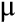and standard deviation, its sample mean,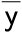, has a sample distribution with the same meanbut whose standard deviation is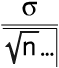.(and we write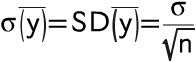).
What do you have to do with quantative data?
Summarize with sans and standard deviations. Think about sampling distributions.
What would be a better term for sampling error?
Sampling variability